Get our FREE VBA eBook of the 30 most useful Excel VBA macros.

Automate Excel so that you can save time and stop doing the jobs a trained monkey could do.

# Why are Excel formulas not calculating?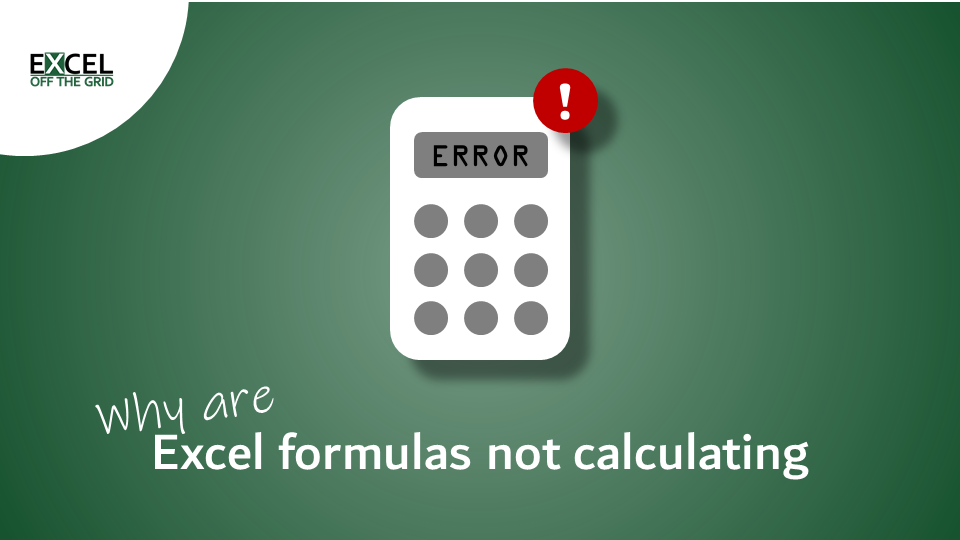We have all experienced it; for whatever reason, the formulas in Excel aren’t calculating as expected. Most of the time, it is something simple that we have never thought of. But, once you know the most likely reasons, it is easier to troubleshoot the problem. So, in this post, we are looking at the most likely reasons for Excel formulas not calculating.

While I would like this to be an exhaustive list, given the depth of Excel, it’s just not possible. However, this should cover most of the issues.

Contents

## Calculation option – automatic or manual

Excel has two calculation options, Automatic and Manual. Most users don’t even know that these two modes exist. Frustratingly, they can also change without us knowing it.

Automatic calculation is the default. This is where formulas recalculate after any change, which affects the result of a calculation.

Excel formulas are efficient at handling calculations; Excel knows which cells impact which formulas. Therefore in Automatic calculation mode, it only recalculates the necessary cells. This means, all the values should be up-to-date. This is the behavior we expect.

However, due to the size and complexity of some spreadsheets or due to 3rd party add-ins, the calculation speed can become very slow. This leads some users to change Excel’s default calculation option to Manual calculation mode. This means Excel doesn’t recalculate anything unless you explicitly tell it to.

If Excel is in manual calculation mode, it would appear that there is an issue because you will see the Excel formula not calculating.

NOTE:

Unfortunately, Excel can change between Manual and Automatic calculation modes without us knowing it. So check out this post: Why does Excel’s calculation mode keep changing?

To find out which calculation mode is enabled, click Formulas > Calculation Options.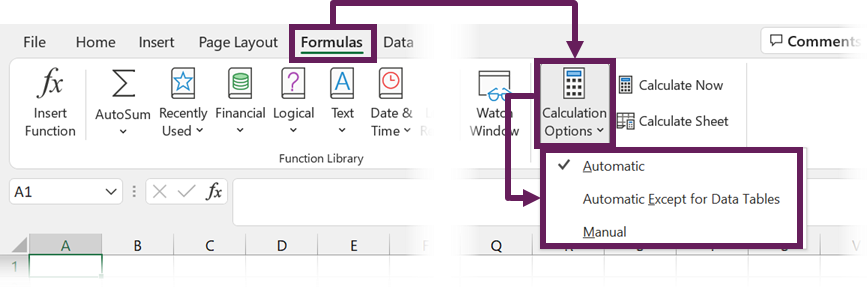Click Automatic to ensure calculation happens automatically.

Calculation mode is an application-level setting; therefore, it applies to all open workbooks.

If you want to remain in Manual calculation mode, there are some useful shortcuts.

• F9: Calculates formulas that have changed since the last calculation, and formulas dependent on them, in all open workbooks.
• Shift + F9: Calculates formulas that have changed since the last calculation, and formulas dependent on them, in the active worksheet.
• Ctrl + Alt + F9: Calculates all formulas in all open workbooks, regardless of whether they have changed since last time or not.
• Ctrl + Shift + Alt + F9: Rechecks dependent formulas and then calculates all formulas in all open workbooks, regardless of whether they have changed since last time or not.

## Wrong number format

When we look at a cell value, we make a decision about what kind of data it is. We know instinctively that numbers can be aggregated, but text cannot. Unfortunately, Excel isn’t so clever.

Within Home > Number we can select the cell format.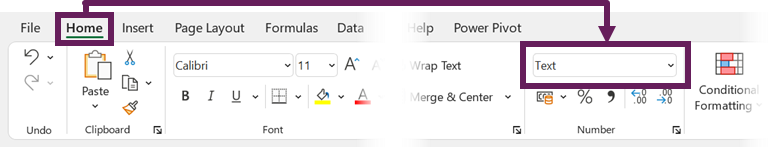If a cell has been formatted as text instead of a number, Excel may not calculate and display the value as we expect.

Look at the screenshot below. The SUM of B2-B3 equals 1; 1+1 = 1 is definitely wrong. This is because B3 has been formatted as text.To fix this, here are 3 options:

1. Manual change
• Change the cell format from Text to another format (General is a good option to start with)
• Double click on the problem cell to go into edit mode
2. If the cell has a green triangle:
• Click on the cell
• Select Convert to number from the warning drop-down3. Multiplying the values by 1:
• Type a 1 into any blank cell.
• Copy the cell with the 1
• Select the cells to be converted to numbers
• Click Home > Paste> Paste Special…
• In the Paste Special window, select Multiply, then click OK

#1 specifically relates to numbers formatted as text, but #2 and #3 can be used in many scenarios where numbers are stored as text.

The apostrophe (‘) is a special character in Excel. Whenever an apostrophe is entered before a value, this tells Excel that everything that follows is text.

This ensures we can store numbers as text. For example, if we wanted to store an employee number that contained leading zeros, Excel would remove the zeros on data entry.

Therefore, we insert the apostrophe to ensure Excel understands this as text.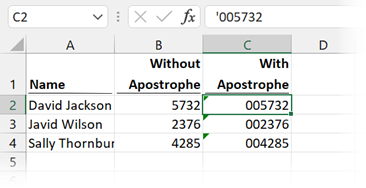However, sometimes this apostrophe can appear when we don’t want it. This often happens when the data is imported from an external system.

The screenshot below shows that the calculation doesn’t work as expected (1 + 1 = 1 is clearly wrong). This is because cell B3 contains a value with an apostrophe preceding it.Either remove the apostrophe manually or use techniques #2 and #3 listed in the section above to fix the issue.

There is a similar impact on formulas. In the screenshot below, the SUM function has a leading apostrophe; therefore, it is treated as text.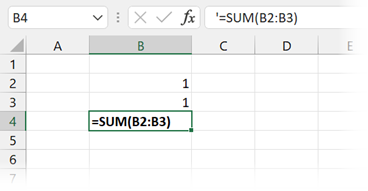As it’s a formula, you will need to remove the apostrophe manually. Then you’re good to go.Leading and trailing spaces are a big problem, they often occur in imported text, but we cannot see them with our eyes.

Let’s look at an example.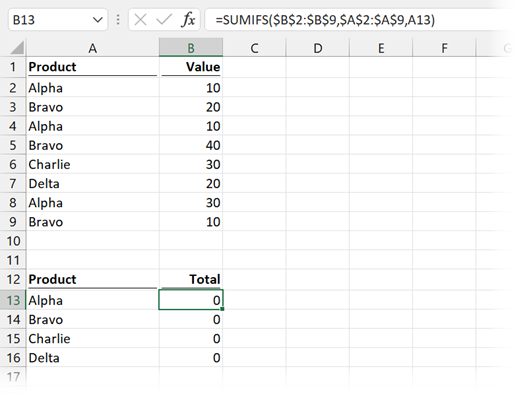In the screenshot above, the formula in Cell B13 is:

`=SUMIFS(\$B\$2:\$B\$9,\$A\$2:\$A\$9,A13)`

We are performing a basic SUMIFS function to add up all the values for product Alpha. Based on the data, the value should be 50, but it is calculating as zero.

The problem here is that all the values in Cells A2:A9 all have trailing spaces. Rather than “Alpha”, used in Cell A13 for the SUMIFS function, the value in the data is “Alpha   ” (with a trailing space). Excel views these as different values.

To fix this, there are lots of options. Some suggestions are:

• Manually removing the space from the data
• Adding the trailing space into our SUMIFS criteria
• Using the TRIM function to remove extra spaces from the start/end of the text
• If there are no spaces contained in the middle of the text, we can use Find and Replace to remove extra spaces.

## Numbers contained in double quotes

Another text/number mix-up issue occurs when numbers are contained in double quotes.

Look at the screenshot below. The scenario is that there is a minimum sale volume of 50; therefore, the value in row 3 has been increased to 50 from 30 using an IF function. But the total at the bottom is incorrect (100 + 50 +100 = 200 is definitely wrong). What’s happened?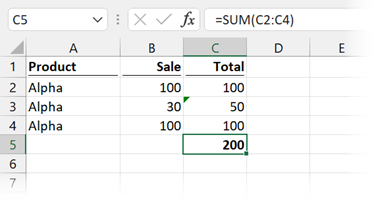The problem is due to a calculation in Cell C3.The formula is:

`=IF(B3<50,"50",B3)`

In this formula, if the value is less than 50, the text value of “50” is returned. The double quotes tell Excel that this is text.

To fix this, remove the double quotes. Then it will return a number.

## Incorrect formula arguments

Functions in Excel are a programming language for calculating a result. Each function has its own syntax (i.e., the arguments required to calculate a result). If we get this syntax wrong, it can lead to unexpected results.

VLOOKUP is a very error-prone formula; it has caught out many unsuspecting users.

Let’s take a look at an example.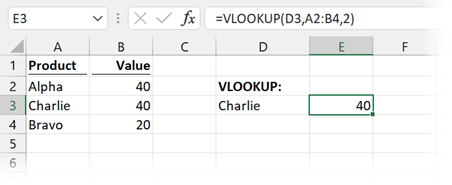In the example above, the formula in Cell E3 is:

`=VLOOKUP(D3,A2:B4,2)`

It has returned a result of 40 for Charlie, which is correct.

Now let’s change the value in D3 to Bravo: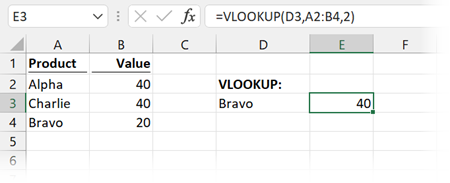It still returns 40!! Bravo should be 20. How is that possible?

In our formula, we excluded the 4th argument, which is the Range_Lookup argument.

• If we enter TRUE or exclude this argument, Excel believes:
• the first column in our data is in sorted ascending order
• we want to return the value which is closest to, but not greater, than the lookup value.
• If we enter FALSE as the fourth argument, Excel will only return an exact match.

As our first column is not in ascending order, Excel has calculated the wrong result.

If we go back and change our formula so the 4th argument is FALSE, it works.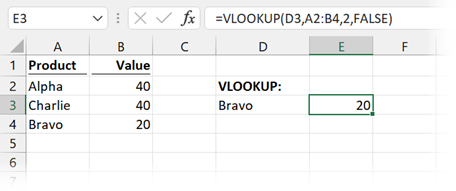This demonstrates the importance of understanding what all function arguments do.

## Non-printed characters

Non-printed characters are letters used within computer code that cannot be viewed by a person. As a simple example, the line break character that we can enter into Excel using Alt + Enter is not a printable character.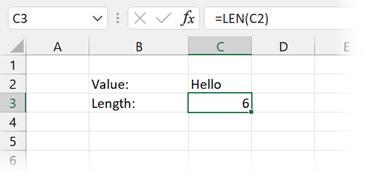In the screenshot above, the LEN function shows that there are 6 characters in Cell C2. But the word “Hello” only has 5 characters.

There are no spaces in Cell C2, so what’s going on? It’s because there is a trailing line break character.

We can remove non-printed characters using the CLEAN function.Look at the screenshot above. Once we’ve used the CLEAN function, the value is now correct again.

## Circular references

Circular references are where a formula refers to a cell that is within its own calculation chain. Here is a basic example.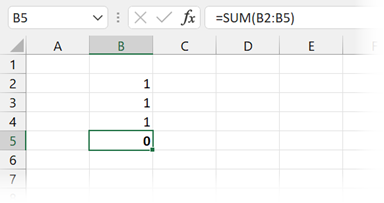The formula in Cell B5 is:

`=SUM(B2:B5)`

If you notice, the cell reference of the formula is included within the range of cells. Excel cannot calculate a result (unless iterative calculations have been enabled).

When we create or open a workbook with circular references, Excel will often display an error message.But, error messages don’t stop us from clicking OK and continuing as if there is nothing wrong.

If there are circular references, they can be tough to find manually. Thankfully, Excel has given us a tool within the Formulas > Formula Auditing > Error Checking > Circular References which displays the circular references in any of our open workbooks.As shown in the screenshot above, Cell B5 has a circular reference. Once we remove that, it will calculate correctly.

## Show formulas

In Excel, we normally view the results of calculations. However, with one button press / or one shortcut, we can quickly toggle to show formulas rather than results.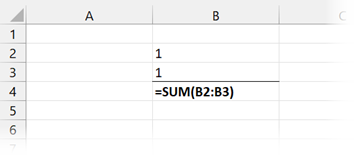Turning on show formulas can easily be performed by accident or saved in this state by a previous user.

To display the formula results again, click Formulas > Formula Auditing> Show Formulas, or using the shortcut key Ctrl + ` (the key to the left of the 1 key on a standard windows keyboard).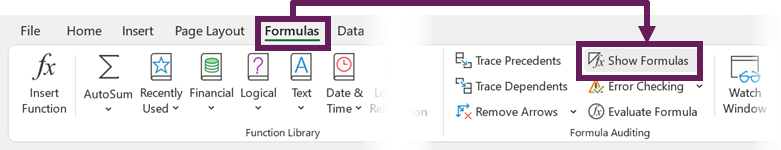## Incorrect cell references

One thing we learn in Excel quite early on is the use of the dollar (\$) symbol to lock cell references. For example:

• If we enter =A1 into a cell and copy it down, it will change to =A2.
• If we enter =A\$1 and copy the cell down, it will remain as =A\$1.

We get used to this referencing syntax quickly. However, this makes it extremely easy to make mistakes when copying formulas to other cells.Look at the screenshot above. There is definitely an issue with the calculation in Cell D6.

Double click on the cell, and the problem will quickly reveal itself.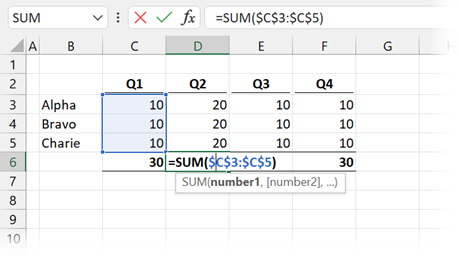Yes… somebody forgot that \$ symbols had been used on Cell C6, then they copied the formula into Cells D6 to F6.

Unfortunately, it’s easily done; I’ve certainly done it myself many times.

How can we find these types of errors? The only way is to check our work as we go, and apply a healthy bit of skepticism to any calculation result. It’s better that you self-review and find it, than to sit in a meeting with your manager and they find the error.

## Automatic data entry conversion

To help reduce the number of errors we make, Excel has been programmed to auto-correct / auto change some data as we enter it. This is great most of the time, and who knows how often it has saved us from an embarrassing mistake.

### Dates

However, the auto-correction can cause problems at other times. For example, if we enter 1/1 into Excel, what do we want? Do we want the result of 1? Or do we want 1st January? In this case, Excel assumes we want 1st January of the current year.

Dates are the most common type of automatic conversion. Dates in Excel are actually numbers, based on the number of days since 31st December 1899. So, 1st January 2021 is the number 44197. We can see this when we change the date to a number format.We tried to enter a value of 1 and ended up with a value of 44197. Hmmm… that could be an issue.

We have already seen above that leading zeros can be a problem.

If we try to enter a number with leading zeros, such as 0005321, Excel will assume we don’t want the zeros and convert it to 5321. To add leading zeros, we should add an apostrophe at the start.

## Hidden rows and columns

This is one of my pet peeves. Hidden rows and columns cause so many problems for Excel users. In my opinion they should be avoided at all costs.

Let’s look at an example: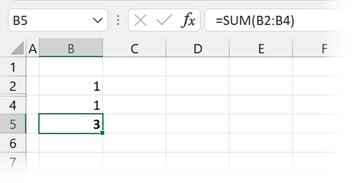Look at the screenshot above. The formula in Cell B5 is:

`=SUM(B2:B4)`

Is this result correct? Actually, yes. But the hidden rows make the result look wrong. The hidden row contains an additional value, as we can see when we unhide row 3.If we want a calculation that excludes hidden cells, then we should consider using the AGGREGATE function.

## Conclusion

So, they are the most likely reasons why we find Excel formula not calculating as expected. I hope these techniques have helped to solve your problem. They should cover the most likely issues.

If you’ve found any other simple issues which cause incorrect calculations, please add them in the comments below.Get our FREE VBA eBook of the 30 most useful Excel VBA macros.

Automate Excel so that you can save time and stop doing the jobs a trained monkey could do.

Don’t forget:

I’m guessing the examples in this post didn’t exactly meet your situation.  We all use Excel differently, so it’s impossible to write a post that will meet everybody’s needs.  By taking the time to understand the techniques and principles in this post (and elsewhere on this site) you should be able to adapt it to your needs.

But, if you’re still struggling you should:

1. Read other blogs, or watch YouTube videos on the same topic.  You will benefit much more by discovering your own solutions.
2. Ask the ‘Excel Ninja’ in your office.  It’s amazing what things other people know.
3. Ask a question in a forum like Mr Excel, or the Microsoft Answers Community.  Remember, the people on these forums are generally giving their time for free.  So take care to craft your question, make sure it’s clear and concise.  List all the things you’ve tried, and provide screenshots, code segments and example workbooks.
4. Use Excel Rescue, who are my consultancy partner.   They help by providing solutions to smaller Excel problems.

What next?
Don’t go yet, there is plenty more to learn on Excel Off The Grid.  Check out the latest posts:

## 10 thoughts on “Why are Excel formulas not calculating?”

1.It is great subject. Such a easy topic but you explained it so many ways. That’s why you are an MS MVP.

•Excel Off The Grid says:

Thanks Adem, I appreciate that 🙂

2.Lebo Baloyi says:

Meged cells are not formula friendly please keep that in mind as well.

•Excel Off The Grid says:

Hi Lebo – Yes, that’s a good addition. Merged cells are a nightmare!

3.Jon Peltier says:

Cleaner convert text to number.

You suggested typing 1, copying this, and paste-special multiplying to the non-numeric cells. This requires the extra steps of getting a cell dirty, then deleting the cell.

Do this instead. Copy a blank cell, then paste-special add it to the non-numeric cells.

•Excel Off The Grid says:

Nice – I had never thought of doing that. So simple, that will save me a couple of clicks 😀

4.Martin R says:

Great synopsis – thanks!

You suggestetd “To add leading zeros, we should add an apostrophe at the start.” A better way since a number should remain a number and not a text is to format the cell with user defined “0000000”

•Excel Off The Grid says:

I partly agree. When a number is a dimension, reference, supplier code, telephone number, credit card number etc, then it will never be aggregated. Therefore text formatting may better reflect the purpose of what the number is. I would take this on a case-by-case basis.

5.Worktime says:

I’ve often gotten a mistake before. Now I understand why. Thanks for the valuable information.

•Excel Off The Grid says:

Good, I’m glad I could help 🙂